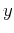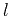Nonhyperbolic reflection moveout of-waves: An overview and comparison of reasonsNext: Conclusions Up: Fomel & Grechka: Nonhyperbolic Previous: Curved reflector beneath homogeneous

# ANISOTROPY VERSUS LATERAL HETEROGENEITY

The nonhyperbolic moveout in homogeneous VTI media with one horizontal reflector is similar to that caused by lateral heterogeneity in isotropic models. In this section, we discuss this similarity following the results of Grechka (1998).

The angle dependence of the group velocity in equations (1) and (5) is characterized by smallanisotropic coefficients. Therefore, we can assume that an analogous influence of lateral heterogeneity might be caused by smallvelocity perturbations. (Large lateral velocity changes can cause behavior too complicated for analytic description.) An appropriate model is a plane laterally heterogeneous layer with the velocity(78)

whereis a dimensionless function. The velocitygiven by equation (78) has the generic perturbation form that allows us to use the tomographic linearization assumption. That is, we neglect the ray bending caused by the small velocity perturbationand compute the perturbation of traveltimes along straight rays in the constant-velocity background. Thus, we can rewrite equation (9) as(79)

whereis the midpoint location and the integration limits correspond to the source and receiver locations. For simplicity and without loss of generality, we can setto zero. Linearizing equation (79) with respect to the small perturbation, we get(80)

It is clear from equation (80) that lateral heterogeneity can cause many different types of the nonhyperbolic moveout. In particular, comparing equations (80) and (11), we conclude that a pseudo-anisotropic behavior of traveltimes is produced by lateral heterogeneity in the form(81)

or, in the linear approximation,(82)

whereandshould be considered now as parameters, describing the isotropiclaterally heterogeneous velocity field. Equation (82) indicates that the velocity heterogeneitythat reproduces moveout (12) in a homogeneous VTI medium, is a symmetric function of the offset. This is not surprising because the velocity function (1), corresponding to vertical transverse isotropy, is symmetric as well.Nonhyperbolic reflection moveout of-waves: An overview and comparison of reasonsNext: Conclusions Up: Fomel & Grechka: Nonhyperbolic Previous: Curved reflector beneath homogeneous

2014-01-27